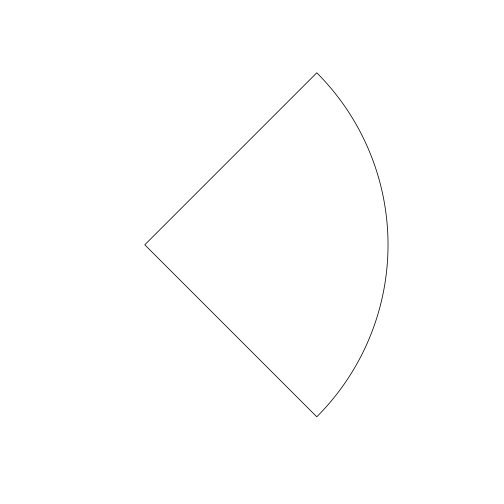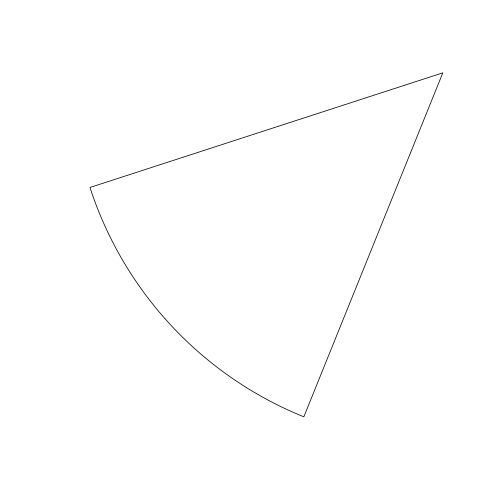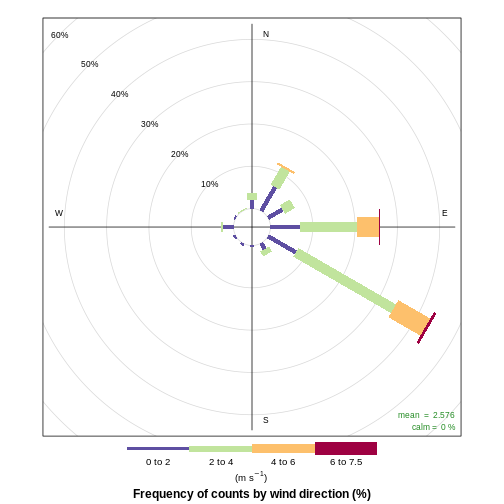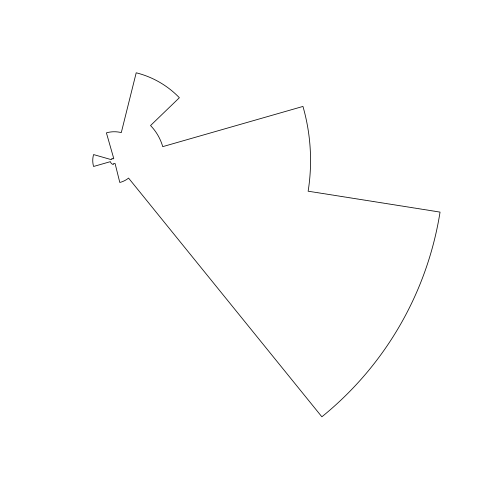### buffeRs basic use

buffeRs will create non-standard buffer geometries around an input point (created by the `sf` package), and has the following functions:

1. `buffer_wedge()` creates a wedge-shaped geometry
2. `buffer_semicircle()` creates a semicircular geometry
3. `buffer_windrose()` creates a windrose shaped geometry
4. `buffer_rectangle()` creates a rectangular shaped geometry
5. `buffer_square()` creates a square shaped geometry

One example would be to build a wedge-shaped buffer with `buffer_wedge()`.

``````library(buffeRs)
example(buffer_wedge)
``````We can also create a different wedge, like this:

``````buffer_wedge(point = example_point, radius = 200, degree = 227, degree_width = 25) -> example_wedge2
plot(example_wedge2)
``````Other geometries are similarly straightfoward, and all functions have parameters to change the radius and the angle of the object.

`buffer_windrose()` is more complex, and requires the input of a wind frequency table, in the format provided by `openair::windRose()\$data`

``````example(buffer_windrose)
``````